## 15 Questions MCQ Test Practice Questions for GMAT | Test: Quadratic Equations- 3

Description
Attempt Test: Quadratic Equations- 3 | 15 questions in 10 minutes | Mock test for GMAT preparation | Free important questions MCQ to study Practice Questions for GMAT for GMAT Exam | Download free PDF with solutions
QUESTION: 1

### A poultry farm has only chickens and pigs. When the manager of the poultry counted the heads of the stock in the farm, the number totaled up to 200. However, when the number of legs was counted, the number totaled up to 540. How many more chickens were there in the farm? Note: In the farm, each pig had 4 legs and each chicken had 2 legs.

Solution:

Let the number of chickens in the farm be 'x'.
Let the number of pigs in the farm be 'y'.

Each pig or each chicken has one head.
Therefore, number of heads will be the same as the sum of the chickens and pigs in the farm.
The count of the heads in the farm is 200. So, the sum of the number of chickens and pigs is 200.
So, x + y = 200 .... (1)

Each chicken has 2 legs and each pig has 4 legs
'x' chickens will therefore, have 2x legs and 'y' pigs will have 4y legs.
The count of the legs in the farm is 540. So, the sum of the number of legs of chickens and the number of legs of pigs is 540.
Therefore, 2x + 4y = 540 .... (2)
Multiply equation (1) by 2. We get 2x + 2y = 400 .... (3)
Subtract equation (3) from equation (2).

2x + 4y = 540
-(2x + 2y = 400)
---------------------------
2y = 140
---------------------------
Or y = 70.

Substitute 'y' as 70 in equation (1).
70 + y = 200. So, x = 130

'x' is the number of chickens in the farm. 'y' is the number of pigs in the farm. So, the farm has 130 chickens and 70 pigs.

Note:The question is "How many more chickens were there in the farm?"
The farm has 130 chickens and 70 pigs. So, the farm has (130 - 70) = 60 more chickens

QUESTION: 2

### Three years back, a father was 24 years older than his son. At present the father is 5 times as old as the son. How old will the son be three years from now?

Solution:

Let the age of the son 3 years back be 'x' years.
Information 1: Three years back, a father was 24 years older than his son.
Therefore, the age of the father 3 years back was (x + 24).

If the age of the son 3 years back was 'x' years, the present age of the son is x + 3.
Present age of father = x + 24 + 3

Information 2: The father is at present 5 times as old as the son.
i.e., (x + 24 + 3) = 5(x + 3)
Or x + 27 = 5x + 15
Or 4x = 12 or x = 3.

x was the age of the son 3 years back.
Therefore, the son was 3 years old 3 years back.

The question is "How old will the son be three years from now?"
If the son was 3 years old, 3 years back, the son is 6 years old now.
Hence, he will be 9 years old three years from now.

QUESTION: 3

### For what values of 'k' will the pair of equations 3x + 4y = 12 and kx + 12y = 30 NOT have a unique solution?

Solution:

A system of linear equations ax + by + c = 0 and dx + ey + g = 0 will have a unique solution if the two lines represented by the equations ax + by + c = 0 and dx + ey + g = 0 intersect at a point.
i.e., if the two lines are neither parallel nor coincident.
Essentially, the slopes of the two lines should be different.

ax + by + c = 0 and dx + ey + g = 0 will intersect at one point if their slopes are different.
Express both the equations in the standardized y = mx + c format, where 'm' is the slope of the line and 'c' is the y-intercept.

ax + by + c = 0 can be written as y =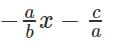And dx + ey + g = 0 can be written as y =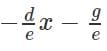Slope of the first line is -a/b and that of the second line is -d/e
For a unique solution, the slopes of the lines should be different.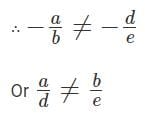The slopes should be equal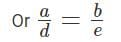Apply the condition in the given equations to find k

In the question given above, a = 3, b = 4, d = k and e = 12.
Therefore,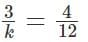Or 'k' should be equal to 9 for the system of linear equations to NOT have a unique solution.

The question is "What is the value of k?
When k = 9, the system of equations will represent a pair of parallel lines (their y-intercepts are different). So, there will be NO solution to this system of linear equations in two variables.

QUESTION: 4

The basic one-way air fare for a child aged between 3 and 10 years costs half the regular fare for an adult plus a reservation charge that is the same on the child's ticket as on the adult's ticket. One reserved ticket for an adult costs \$216 and the cost of a reserved ticket for an adult and a child (aged between 3 and 10) costs \$327. What is the basic fare for the journey for an adult?

Solution:

Let the basic fare for the child be \$X.

Information 1: Basic one-way air fare of a child costs half the regular fare for an adult.
Therefore, the basic fare for an adult = 2(basic one-way airfare for a child) = \$2X.

Information 2: Reservation charge is the same on the child's ticket as on the adult's ticket.
Let the reservation charge per ticket be \$Y
A child's ticket will cost (Basic fare + Reservation charges) = X + Y
Hence, an adult ticket will cost (Basic fare + Reservation charges) = 2X + Y.
Information 3: One reserved ticket for an adult costs \$216. So, 2X + Y = \$216 .... (1)
Information 4: The cost of a reserved ticket for an adult and a child (aged between 3 and 10) is \$327.
So, the ticket for an adult and a child will cost (2X + Y) + (X + Y) = 3X + 2Y = \$327 .... (2)
Multiply equation (1) by 2: 4X + 2Y = 432 .... (3)
Subtract equation (2) from equation (3):
4X + 2Y = 432
- (3X + 2Y = 327)
------------------------------
X = \$105
------------------------------
The question is "What is the basic fare for an adult?"
The basic fare of an adult ticket = 2X = 2*105 = \$210

QUESTION: 5

Numbers: All numbers used are real numbers.

Figures: A figure accompanying a data sufficiency question will conform to the information given in the question but will not necessarily conform to the additional information given in statements (1) and (2)

Lines shown as straight can be assumed to be straight and lines that appear jagged can also be assumed to be straight
You may assume that the positions of points, angles, regions, etc. exist in the order shown and that angle measures are greater than zero.
All figures lie in a plane unless otherwise indicated.
Q.
Is y = 3?

1. (y - 3)(x - 4) = 0
2. (x - 4) = 0
Solution:

Evaluate Statement (1) ALONE: (y - 3)(x - 4) = 0
If product of the two terms (y - 3) and (x - 4) is 0, then at least one of the two terms equals 0.
(y - 3) = 0 or (x - 4) = 0 or both (y - 3) and (x - 4) equal 0.
i.e., either y = 3 or x = 4 or that both y = 3 and x = 4.
If x = 4, y could take any value. The value of 'y' could be 3 or it could be some other value and the product will still be a 0.
Example: x = 4 and y = 5. The equation holds good. y ≠ 3.
Counter example: x = 4 and y = 3. The equation holds good. y = 3
We CANNOT determine whether 'y' is 3 from this statement.

Eliminate choices A and D. Choices narrow down to B, C or E.
Evaluate Statement (2) ALONE: (x - 4) = 0
The statement provides no information about y.
Evaluate Statements (1) & (2) Together: (y - 3)(x - 4) = 0 & (x - 4) = 0
When x = 4, (y - 3)(x - 4) will be 0 irrespective of the value that y takes.
Yes 'y' can be 3.
Eliminate choice C.

QUESTION: 6

A children's gift store sells gift certificates in denominations of \$3 and \$5. The store sold 'm' \$3 certificates and 'n' \$5 certificates worth \$93 on a Saturday afternoon. If 'm' and 'n' are natural numbers, how many different values can 'm' take?

Solution:

Understand Key Data
Total value of all certificates sold = \$93.
Certificates sold were in denominations of \$3 and \$5.
Both 'm' and 'n' are natural numbers.

The value of all certificates sold, 93 is divisible by 3.
So, a maximum of 31 \$3 certificates and no \$5 certificates could have been sold.
However, the question states that both 'm' and 'n' are natural numbers.
Hence, at least 1 \$5 certificate should have been sold.

Let us reduce the number of \$3 certificates from theoretical maximum count of 31 by say 'x' and correspondingly increase \$5 certificates by 'y'.
Evidently, 3x = 5y because the value of \$3 certificates reduced should be the same as the value of \$5 certificates increased.
It means that x has to be a multiple of 5 and y has to be a multiple of 3.
Or \$3 certificates reduce in steps of 5 certificates.

List down possible values for 'm' and 'n'
The following combinations are possible.

m = 26, n = 3
m = 21, n = 6
m = 16, n = 9
m = 11, n = 12
m = 6, n = 15
m = 1, n = 18
The question is "How many different values can 'm' take?"
Hence, m can take 6 values.

Choice C is the correct answer.

QUESTION: 7

What is the highest integral value of 'k' for which the quadratic equation x2 - 6x + k = 0 will have two real and distinct roots?

Solution:

Nature of Roots of Quadratic Equation Theory
D is called the Discriminant of a quadratic equation.
D = b2 - 4ac for quadratic equations of the form ax2 + bx + c = 0.
If D > 0, roots of such quadratic equations are Real and Distinct
If D = 0, roots of such quadratic equations are Real and Equal
If D < 0, roots of such quadratic equations are Imaginary. i.e., such quadratic equations will NOT have real roots.
In this GMAT sample question in algebra, we have to determine the largest integer value that ‘k’ can take such that the discriminant of the quadratic equation is positive.

Compute maximum value of k that will make the discriminant of the quadratic equation positive
The given quadratic equation is x2 - 6x + k = 0.
In this equation, a = 1, b = -6 and c = k
The value of the discriminant, D = 62 - 4 * 1 * k
We have compute values of 'k' for which D > 0.
i.e., 36 – 4k > 0
Or 36 > 4k or k < 9.

The question is "What is the highest integer value that k can take?"
If k < 9, the highest integer value that k can take is 8.
Choice D is the correct answer.

QUESTION: 8

If one of the roots of the quadratic equation x2 + mx + 24 = 0 is 1.5, then what is the value of m?

Solution:

The given quadratic equation is x2 + mx + 24 = 0
Substitute x = 1.5 in the above equation because 1.5 is a root of the equation.
(1.5)2 + 1.5m + 24 = 0
2.25 + 1.5m + 24 = 0
1.5m = -26.25 Or m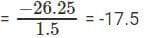QUESTION: 9

For what value of 'm' will the quadratic equation x2 - mx + 4 = 0 have real and equal roots?

Solution:

D is the Discriminant in a quadratic equation.
D = b2 - 4ac for quadratic equations of the form ax2 + bx + c = 0.
If D > 0, roots are Real and Unique (Distinct and real roots).
If D = 0, roots are Real and Equal.
If D < 0, roots are Imaginary. The roots of such quadratic equations are NOT real.
The quadratic equation given in this question has real and equal roots. Therefore, its discriminant D = 0.
In the given equation x2 - mx + 4 = 0, a = 1, b = -m and c = 4.
Therefore, the discriminant b2 - 4ac = m2 - 4(4)(1) = m2 - 16.
The roots of the given equation are real and equal.
Therefore, m2 - 16 = 0 or m2 = 16 or m = +4 or m = -4.

QUESTION: 10

y = x2 + bx + 256 cuts the x axis at (h, 0) and (k, 0). If h and k are integers, what is the least value of b?

Solution:

The given equation is a quadratic equation. A quadratic equation when plotted on a graph sheet (x - y plane) will result in a parabola.
The roots of the quadratic equation are computed by equating the quadratic expression to 0. i.e., the roots are the values that 'x' take when y = 0
So, the roots of the quadratic equation are the points where the parabola cuts the x-axis.
The question mentions that the curve described by the equation cuts the x-axis at (h, 0) and (k, 0). So, h and k are the roots of the quadratic equation.
For quadratic equations of the form ax2 + bx + c = 0, the sum of the roots = -b/a
The sum of the roots of this equation is -b/1 = -b
Note : Higher the value of 'b', i.e., higher the sum of the roots of this quadratic equation, lower the value of b.
For quadratic equations of the form ax2 + bx + c = 0, the product of roots = c/a
Therefore, the product of the roots of this equation = 256/1 = 256
i.e., h * k = 256 h and k are both integers.
So, h and k are both integral factors of 256.

This is the step in which number properties kicks in. 256 can be expressed as product of two numbers in the following ways:
1 * 256
2 * 128
4 * 64
8 * 32
16 * 16

The sum of the roots is maximum when the roots are 1 and 256 and the maximum sum is 1 + 256 = 257.
∴ The least value possible for b is -257.

QUESTION: 11

x2 + bx + 72 = 0 has two distinct integer roots; how many values are possible for 'b'?

Solution:

In quadratic equations of the form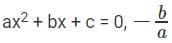represents the sum of the roots of the quadratic equation and c/a  represents the product of the roots of the quadratic equation.
In the equation given a = 1, b = b and c = 72
So, the product of roots of the quadratic equation = 72/1
And the sum of roots of this quadratic equation = -b/1 = -b

We have been asked to find the number of values that 'b' can take.
If we list all possible combinations for the roots of the quadratic equation, we can find out the number of values the sum of the roots of the quadratic equation can take.
Consequently, we will be able to find the number of values that 'b' can take.

The question states that the roots are integers.
If the roots are r1 and r2, then r1 * r2 = 72, where both r1 and r2 are integers.
Possible combinations of integers whose product equal 72 are : (1, 72), (2, 36), (3, 24), (4, 18), (6, 12) and (8, 9) where both r1 and r2 are positive. 6 combinations.

For each of these combinations, both r1 and r2 could be negative and their product will still be 72.
i.e., r1 and r2 can take the following values too : (-1, -72), (-2, -36), (-3, -24), (-4, -18), (-6, -12) and (-8, -9). 6 combinations.
Therefore, 12 combinations are possible where the product of r1 and r2 is 72.
Hence, 'b' will take 12 possible values.

QUESTION: 12

If x > 0, how many integer values of (x, y) will satisfy the equation 5x + 4|y| = 55?

Solution:

5x + 4|y| = 55
The equation can be rewritten as 4|y| = 55 - 5x.
Because |y| is non-negative, 4|y| will be non-negative. Therefore, (55 - 5x) cannot take negative values.

Because x and y are integers, 4|y| will be a multiple of 4.
Therefore, (55 - 5x) will also be a multiple of 4.
55 is a multiple of 5. 5x is a multiple of 5 for integer x. So, 55 - 5x will always be a multiple of 5 for any integer value of x.
So, 55 - 5x will be a multiple of 4 and 5.
i.e., 55 - 5x will be a multiple of 20.

Integer values of x > 0 that will satisfy the condition that (55 - 5x) is a multiple of 20:
1. x = 3, 55 - 5x = 55 - 15 = 40.
2. x = 7, 55 - 5x = 55 - 35 = 20
3. x = 11, 55 - 5x = 55 - 55 = 0.
When x = 15, (55 - 5x) = (55 - 75) = -20. Because (55 - 5x) has to non-negative x = 15 or values greater than 15 are not possible.
So, x can take only 3 values viz., 3, 7, and 11.

We have 3 possible values for 55 - 5x. So, we will have these 3 values possible for 4|y|.
Possibility 1: 4|y| = 40 or |y| = 10. So, y = 10 or -10.
Possibility 2: 4|y| = 20 or |y| = 5. So, y = 5 or -5.
Possibility 3: 4|y| = 0 or |y| = 0. So, y = 0.

Number of values possible for y = 5.

The correct choice is (C) and the correct answer is 5.

QUESTION: 13

If p > 0, and x2 - 11x + p = 0 has integer roots, how many integer values can 'p' take?

Solution:

The given quadratic equation is x2 - 11x + p = 0.
The sum of the roots of this quadratic equation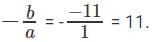The product of the roots of this quadratic equation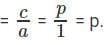The question states that p > 0.
'p' is the product of the roots of this quadratic equation. So, the product of the two roots is positive.
The product of two numbers is positive if either both the numbers are positive or both the numbers are negative.
We also know that the sum of the roots = 11, which is positive.
The sum of two negative numbers cannot be positive.
So, both the roots have to be positive.

We also know that the roots are integers.
So, we have to find different ways of expressing 11 as a sum of two positive integers.
Possibility 1: (1, 10)
Possibility 2: (2, 9)
Possibility 3: (3, 8)
Possibility 4: (4, 7)
Possibility 5: (5, 6)

Each of these pairs, will result in a different value for p.
So, p can take 5 different values.

The correct choice is (C) and the correct answer is 5.

QUESTION: 14

How many real solutions exist for the equation x2 – 11|x| - 60 = 0?

Solution:

Let |x| = y.
We can rewrite the equation x2 - 11|x| - 60 = 0 as y2 - 11y - 60 = 0
The equation can be factorized as y2 - 15y + 4y - 60 = 0
(y - 15) (y + 4) = 0
The values of y that satisfy the equation are y = 15 or y = -4.

We have assigned y = |x|
|x| is always a non-negative number.
So, |x| cannot be -4.
|x| can take only one value = 15.
If |x| = 15, x = 15 or -15.

The number of real solutions that exist for x2 – 11|x| - 60 = 0 is 2.
The correct choice is (B) and the correct answer is 2

QUESTION: 15

If the curve described by the equation y = x2 + bx + c cuts the x-axis at -4 and y axis at 4, at which other point does it cut the x-axis?

Solution:

y = x2 + bx + c is a quadratic equation and the equation represents a parabola.
The curve cuts the y axis at 4.
The x coordinate of the point where it cuts the y axis = 0.
Therefore, (0, 4) is a point on the curve and will satisfy the equation.
Substitute y = 4 and x = 0 in the quadratic equation: 4 = 02 + b(0) + c
Or c = 4.
The product of the roots of a quadratic equation is c/a
In this question, the product of the roots = 4/1 = 4
The roots of a quadratic equation are the points where the curve (parabola) cuts the x-axis.
The question states that one of the points where the curve cuts the x-axis is -4.
So, -4 is one of roots of the quadratic equation.
Let the second root of the quadratic equation be r2.
From step 2, we know that the product of the roots of this quadratic equation is 4.
So, -4 * r2 = 4
or r2 = -1.
The second root is the second point where the curve cuts the x-axis, which is -1.
The correct choice is (A) and the correct answer is -1.Use Code STAYHOME200 and get INR 200 additional OFF Use Coupon Code